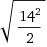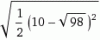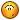BrainDen.com - Brain Teasers

## Question

Two hallways, of widths 4 and 6 feet, meet at a corner. You and a friend are borrowing a 14-foot ladder and need to get it past the corner. If the ends of the ladder are kept at the same height, is it possible to carry the ladder from one hallway to the next? What is the longest ladder that can pass?

## Recommended Posts

• 0

If x is the acute angle formed between the ladder and the outside wall
of the 4 foot corridor, then the longest ladder to form it will be
(4/sin x)+(6/cos x). This is maximal at x=arctan((2/3)^(1/3)). The longest
ladder, therefore, has length approximately 14.047 feet. So, the 14 foot

##### Share on other sites

• 0

If I'm reading this right, the longest possible ladder to fit through the passageway would touch both outer walls of each hallway at a 45 degree angle and will also touch the corner where the hallways meet. This would form two right triangles, and the ladder would form the hypotenuse of both of them. Since the other two angles in each right triangle are both 45 degrees, then the non-hypotenuse sides (forgot what they were called) in each triangle would be the same as well. So the total length of the longest ladder would be= 14.14213562373...

So yes, the 14 foot ladder should pass through from one hallway to the other.

• 0

12.8

Edited by jhawk
##### Share on other sites

• 0

If I'm reading this right, the longest possible ladder to fit through the passageway would touch both outer walls of each hallway at a 45 degree angle and will also touch the corner where the hallways meet. This would form two right triangles, and the ladder would form the hypotenuse of both of them. Since the other two angles in each right triangle are both 45 degrees, then the non-hypotenuse sides (forgot what they were called) in each triangle would be the same as well. So the total length of the longest ladder would be= 14.14213562373...

So yes, the 14 foot ladder should pass through from one hallway to the other.

Of course, that doesn't factor in the thickness of the ladder.

The ladder as a whole would act as the hypotenuse of right triangle with each outer hallway wall. For a 0 width ladder, the length of each wall segment would be= 10. For a 14-foot ladder, they would each be= 9.89949...

If I'm mathing correctly, the difference between these two side lengths should be the hypotenuse of another right triangle, where the other two sides are equal to the maximum thickness of the 14-foot ladder.= 0.0710678...

So for a 14-foot ladder, if it is thinner than 0.0710678... feet it would pass, but if it was thicker it would not pass.

##### Share on other sites

• 0

I've been lurking here. for once let me postnot elegant, but i think it's correct

If the ladder is touching the corner at an angle α on the 6' hallway.

the part of the ladder in the 6' Halway is 6'/cosα

the part of the ladder in the 4' Halway is 4'/sinα

Total length is L=6'/cosα + 4'/sinα

dL/dα= - 6sinα/cos²α + 4cosα/sin²α = (4cos³α-6sin³α)/(sin²α . cos²α )

the minimum is thus where (4cos³α-6sin³α)=0 which resolve to α=atan( squarecube(4/6) ) = 41.14 degree

so the maximum length is L(41.14)=14.047'

the general solution for A and B hallways is L=A/cosα + B/sinα with α=atan( squarecube(B/A) )

##### Share on other sites

• 0

SP and Xavier both have it. SP by a nose. Nice solve both.

Xavier, welcome to active duty!

BobbyGo made an assumption which only holds when the halls are of equal widths,

But provided a maximum ladder thickness as well.

## Join the conversation

You can post now and register later. If you have an account, sign in now to post with your account.×   Pasted as rich text.   Paste as plain text instead

Only 75 emoji are allowed.

×   Your previous content has been restored.   Clear editor

×   You cannot paste images directly. Upload or insert images from URL.